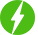# Differential Equations

1

Differential Equations, First Order First Degree Differential Equation: Exact & Linear Differential Equation & Bernoulli’s Differential Equation.

## Course Description

This course has everything you need to learn and understand Differential Equations. Differential equations are a class of equation that involves the use of differentials (derivatives) in their construction. Differential equation arise in a various applications such as velocity, accelerations, mechanical, electrical systems, conduction of heat. Differential equations are used in many areas of science, particularly in physics, where they are used to model real-world phenomena such as the propagation of waves.

Differential equations are also ised i modeling of day to day life situations like concentration of traffic on roads in urban areas, arrival of customers in shoping mauls, landing of planes at the crowded airpors and so on.

If an estimation of quantity depends upon only one factor, we come across situation of one dependent variable and one independent variable and modeling of such phenomena will involve ordinary differential equation.

This course covers:

• Concept of Ordinary Differential Equation
• Order & Degree of Ordinary Differential Equation
• First Order First Degree Differential Equation
• General & Particular Solution of Differential Equation
• Variable Separable Form
• Homogeneous Differential Equation
• Exact Differential Equation
• Reducible To Exact Differential Equation
• Linear Differential Equation
• Reducible To Linear Differential Equation
• Bernoulli’s Differential Equation

Course pre-requisites:

1. Fundamental understanding of differentiation and integration
2. Knowledge of common integration operations (integration by parts, integration by substitution etc.

We will be happy to hear your thoughtsRegister New Account
• Total (0)
0